# Selina Solutions Concise Maths Class 10 Chapter 18 Tangents and Intersecting Chords Exercise 18(C)

This exercise deals with problems based on all the concepts of the chapter. These exercise problems can be effectively solved with guidance of the Selina Solutions for Class 10 Maths. It is also a wonderful resource for students to clarify their doubts and boost the exam preparations. The solutions of the Concise Selina Solutions for Class 10 Maths Chapter 18 Tangents and Intersecting Chords Exercise 18(C) are available in a PDF, which is provided in the link given below.

## Selina Solutions Concise Maths Class 10 Chapter 18 Tangents and Intersecting Chords Exercise 18(C) Download PDF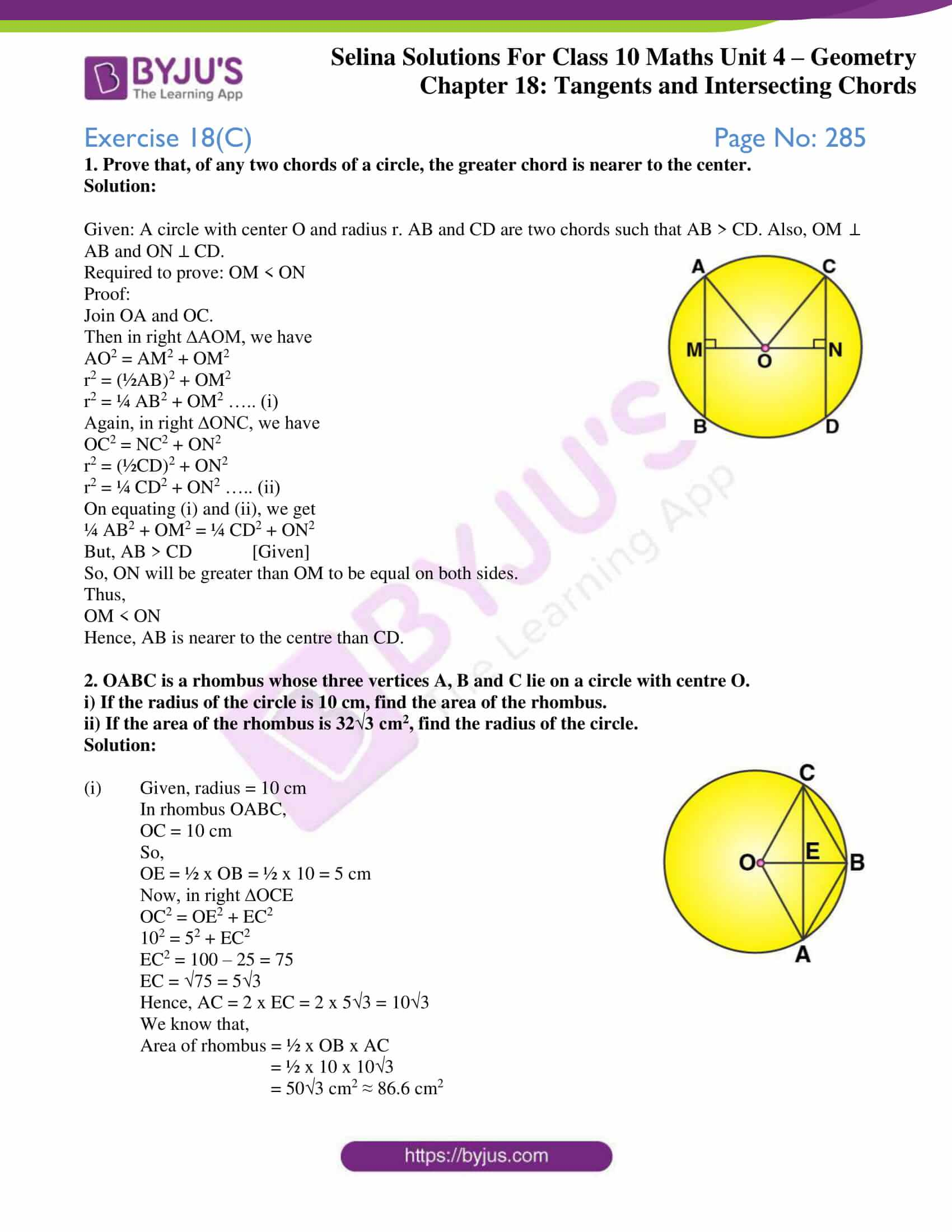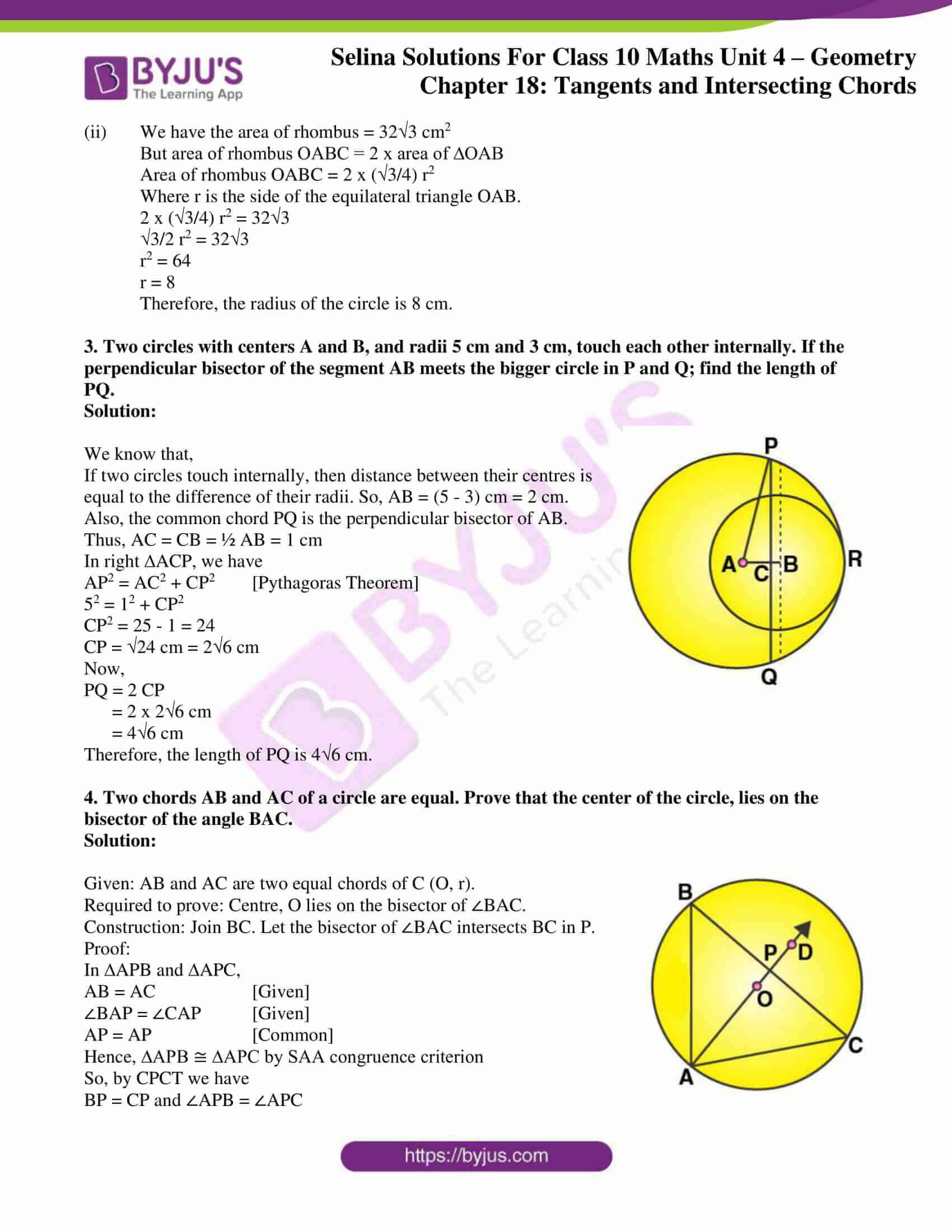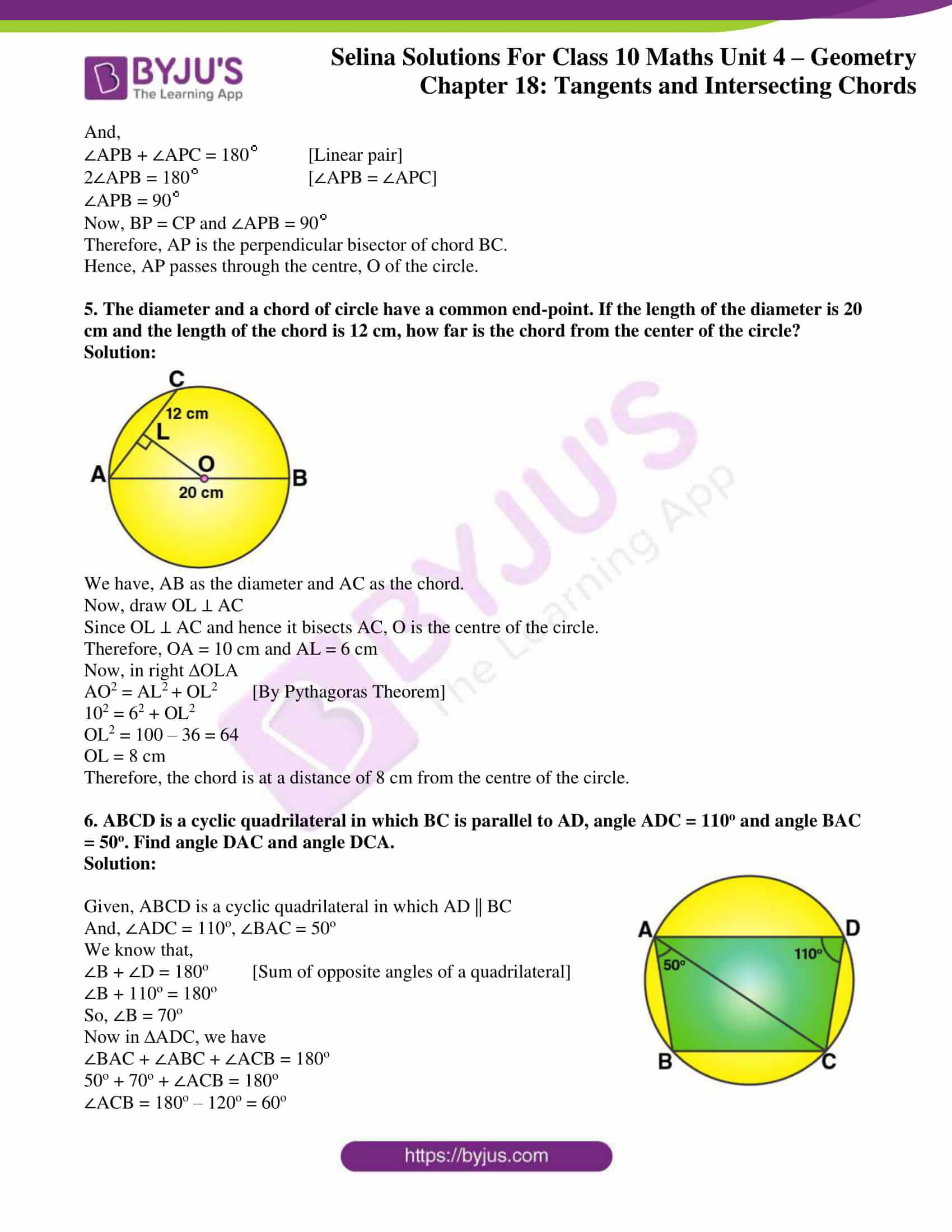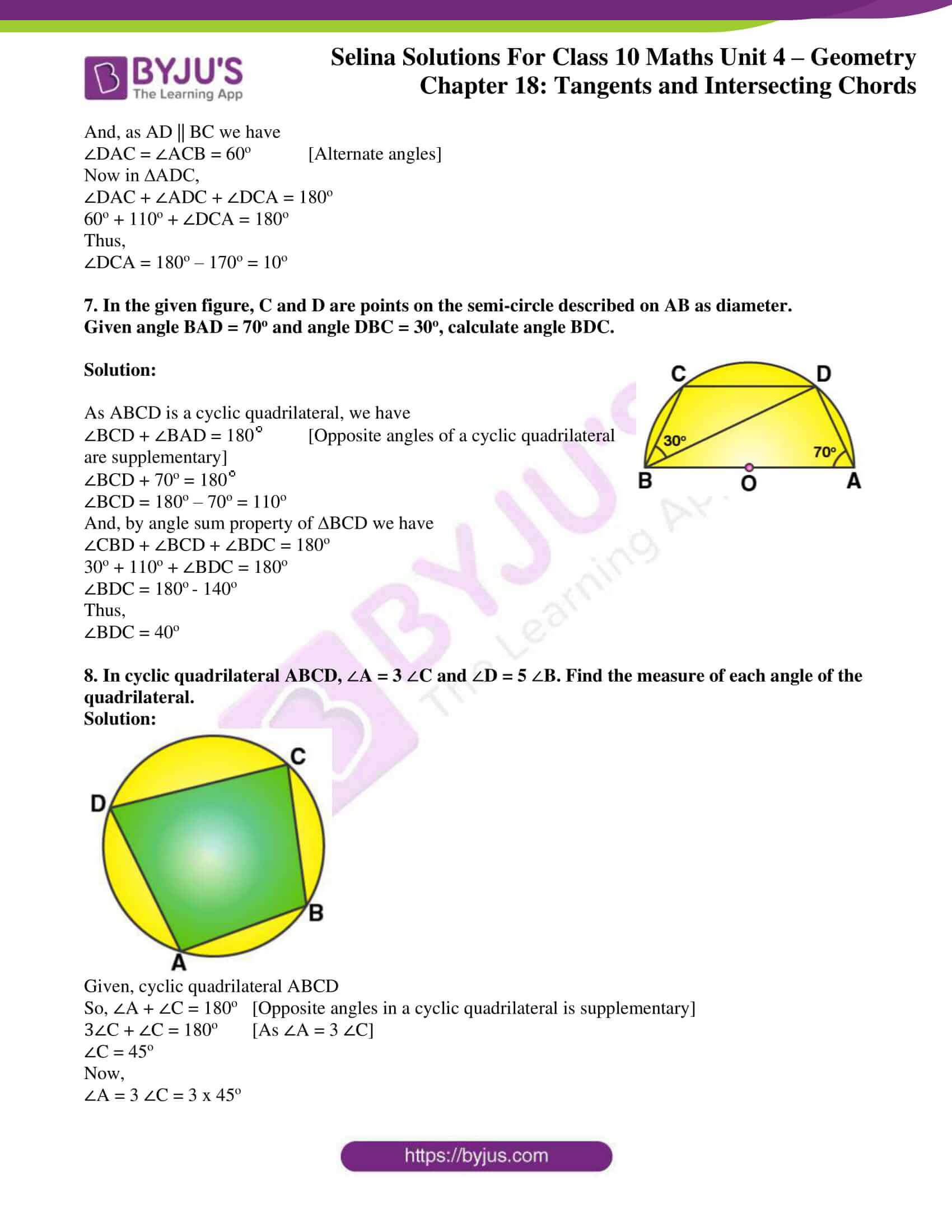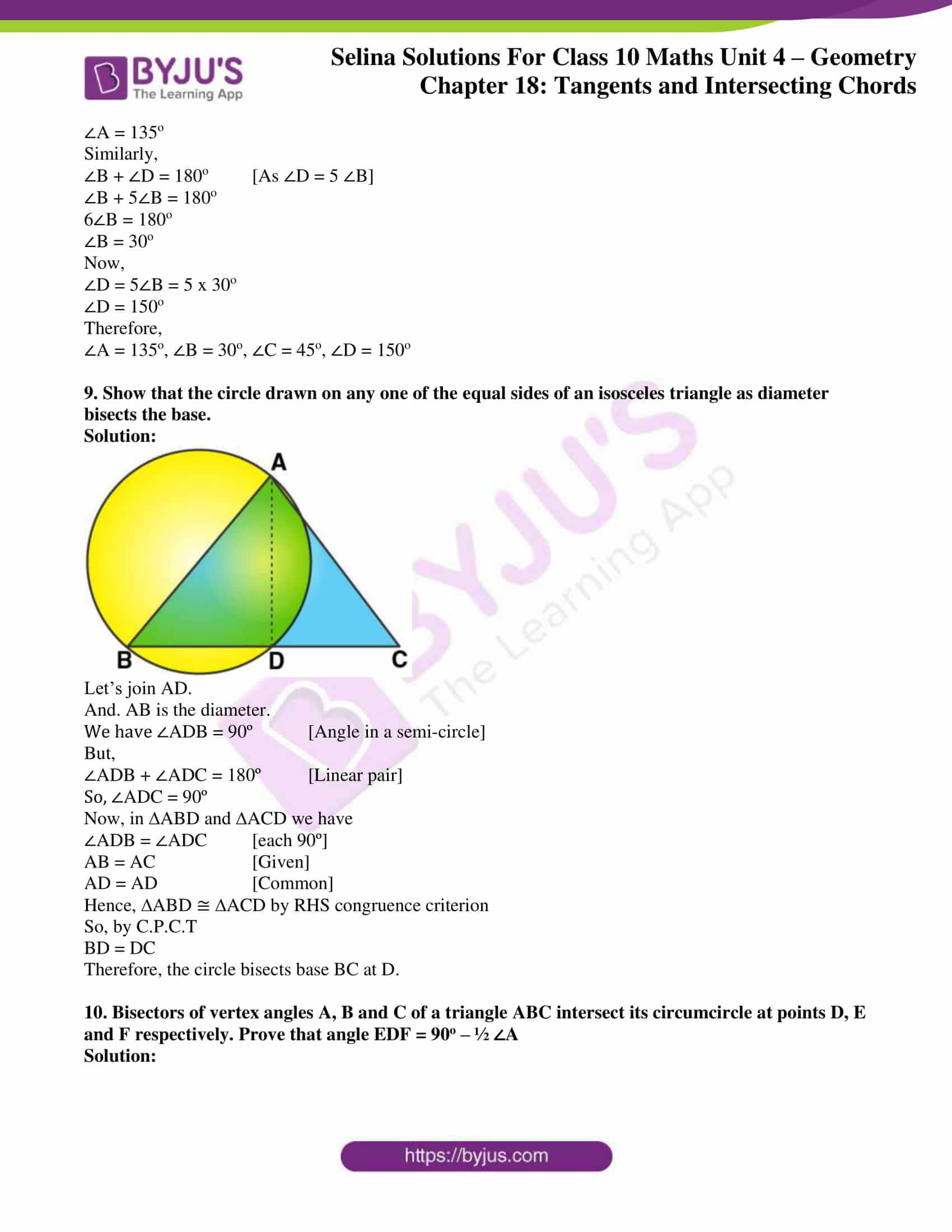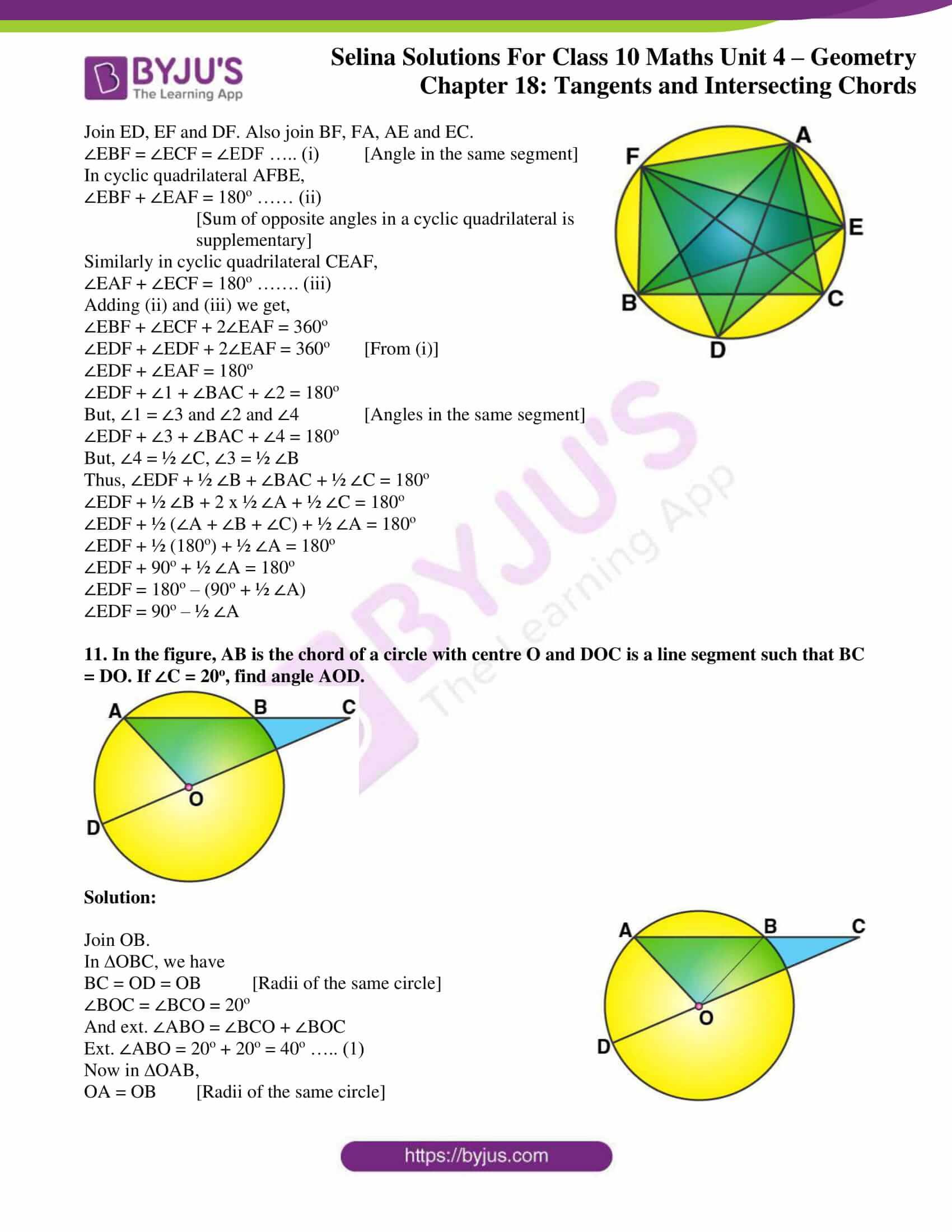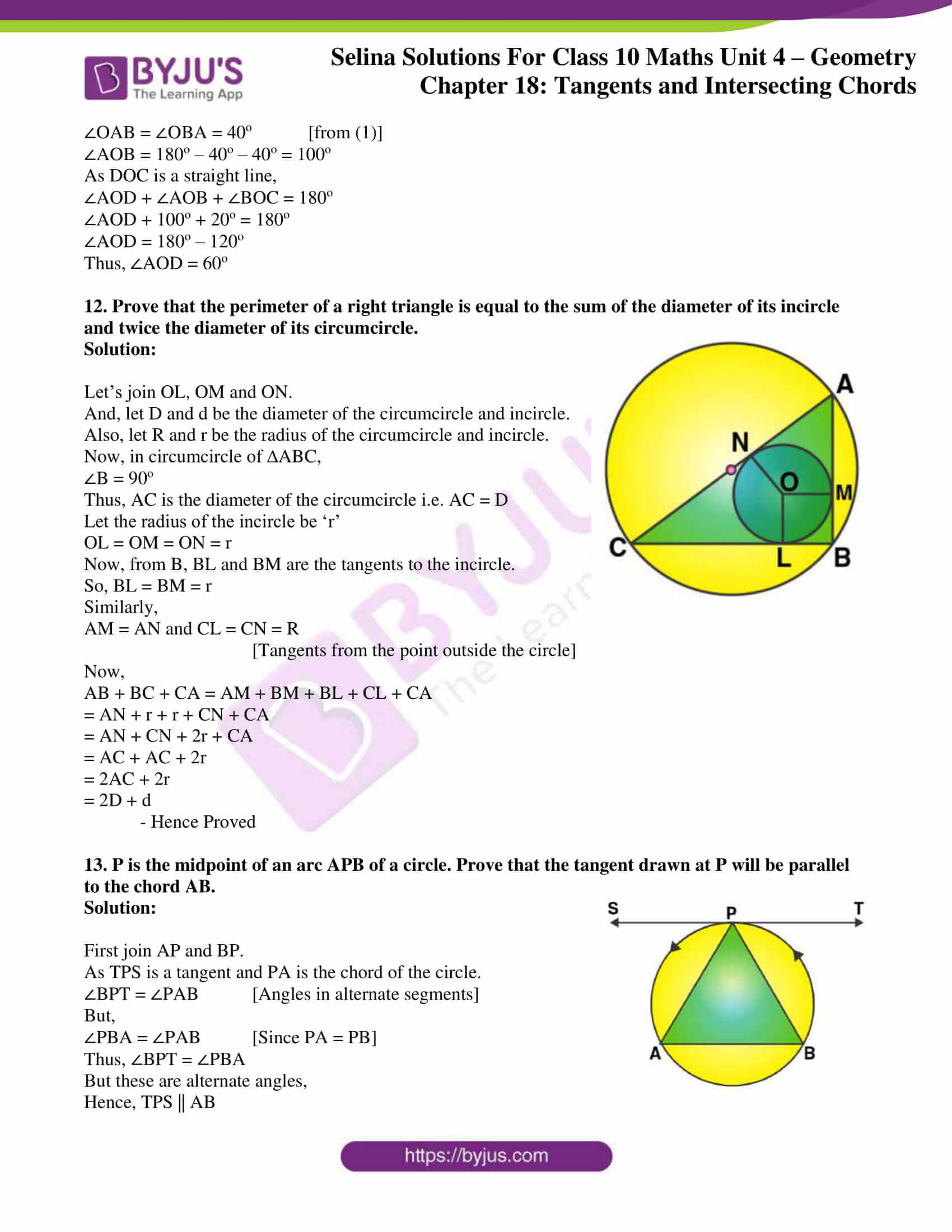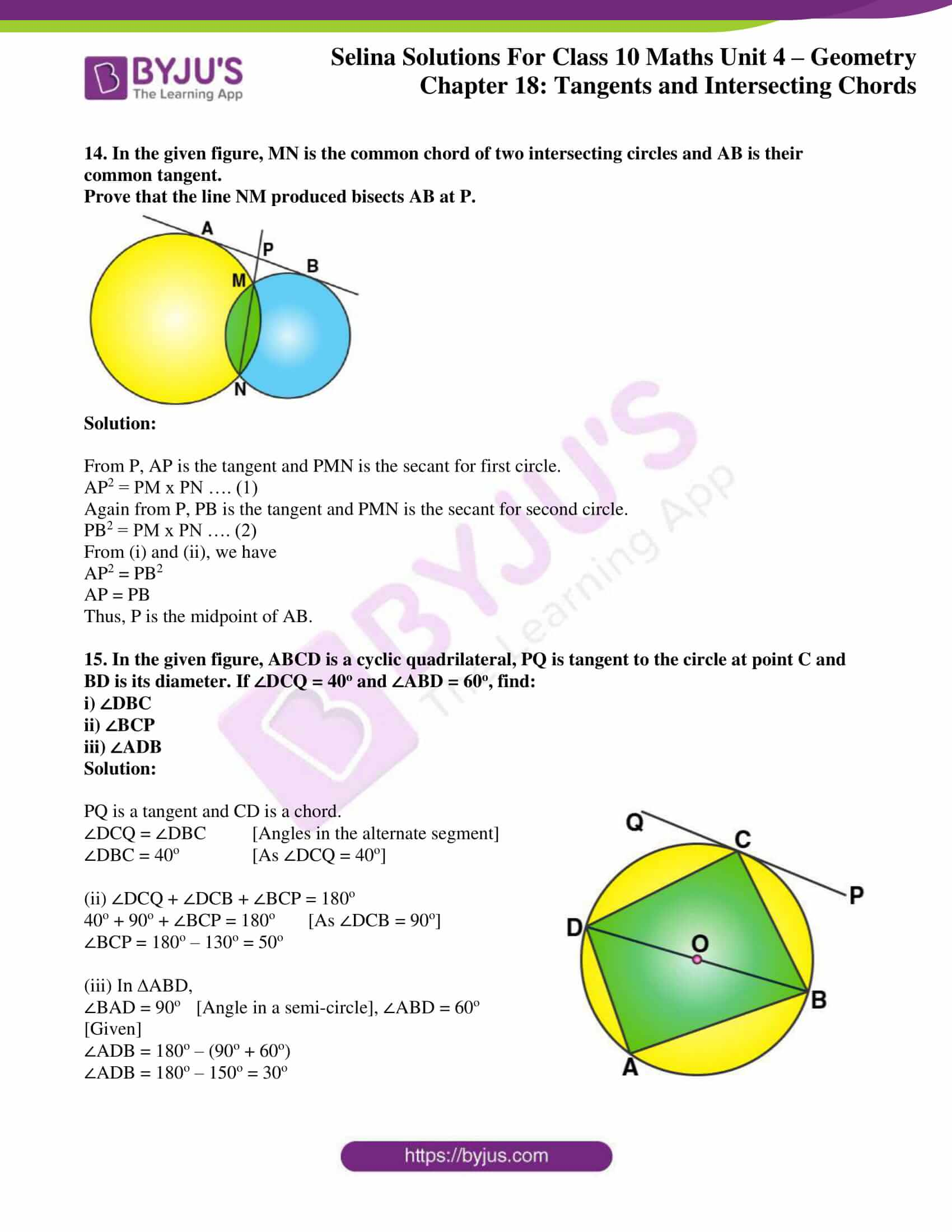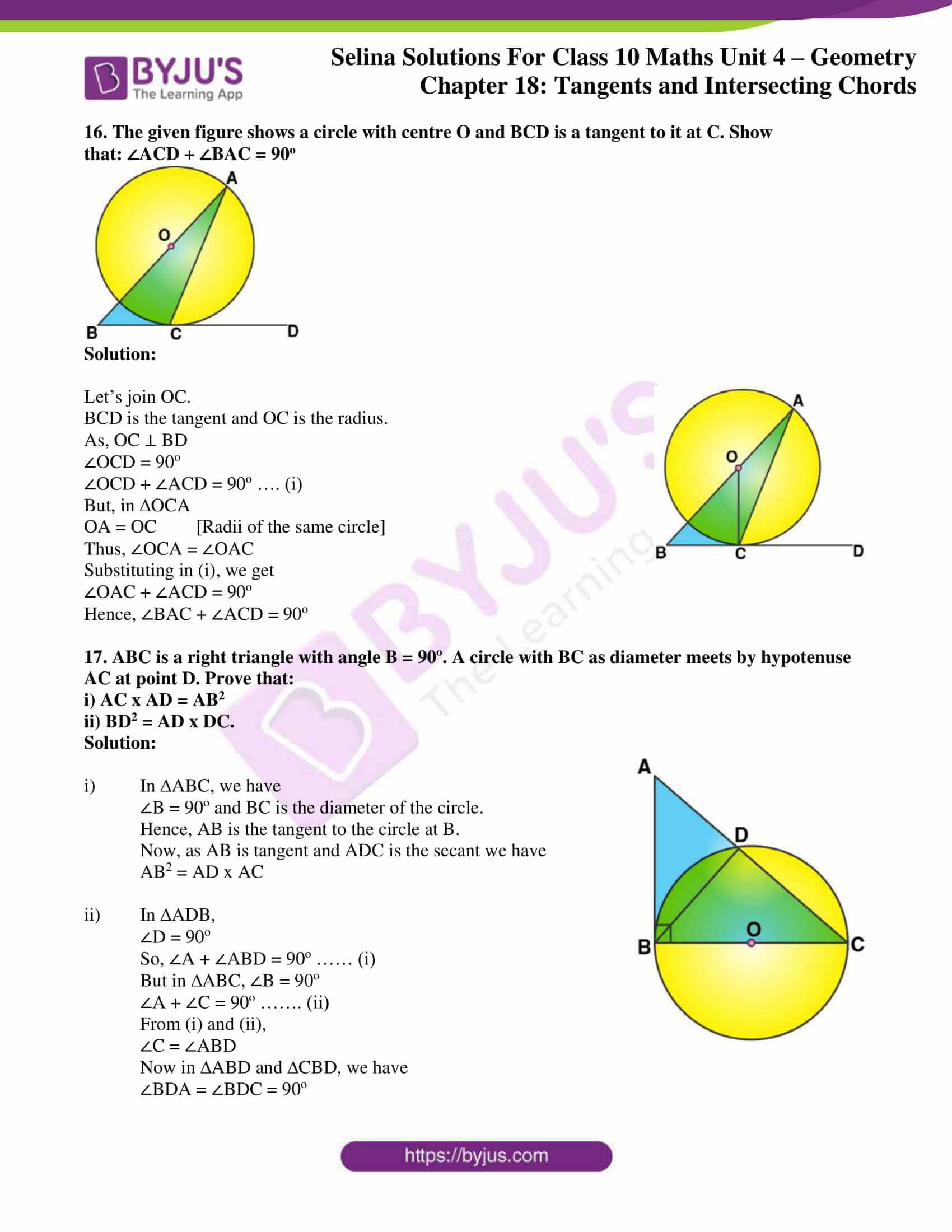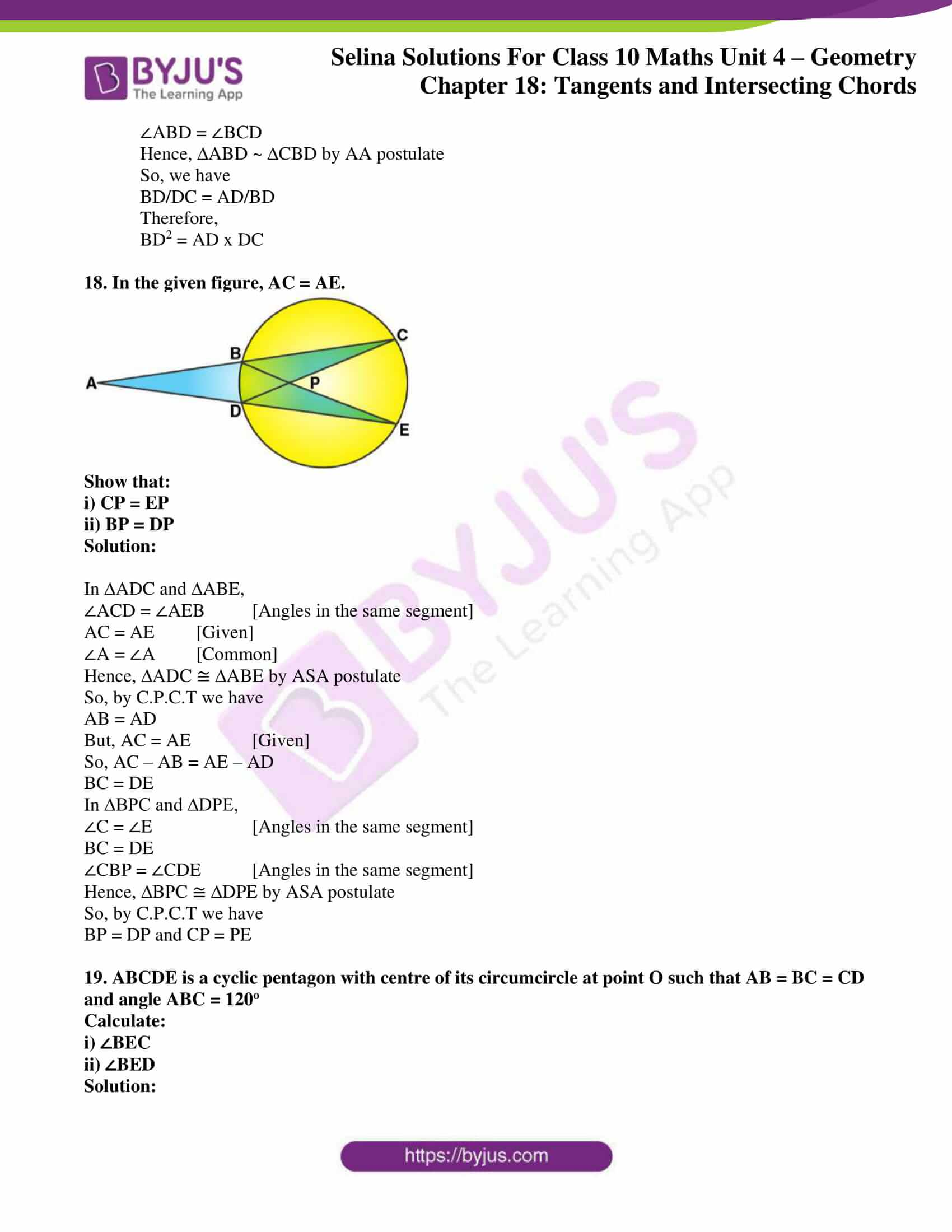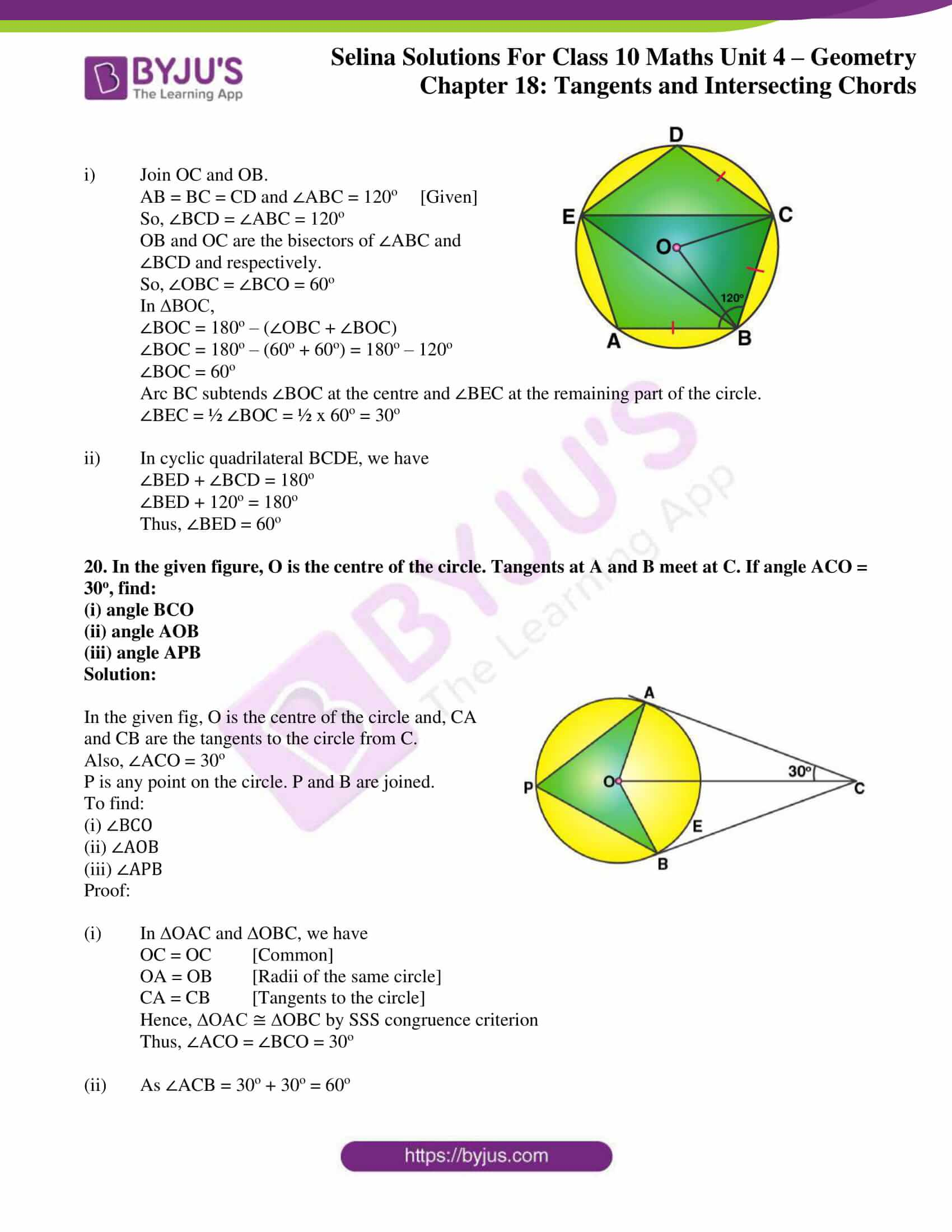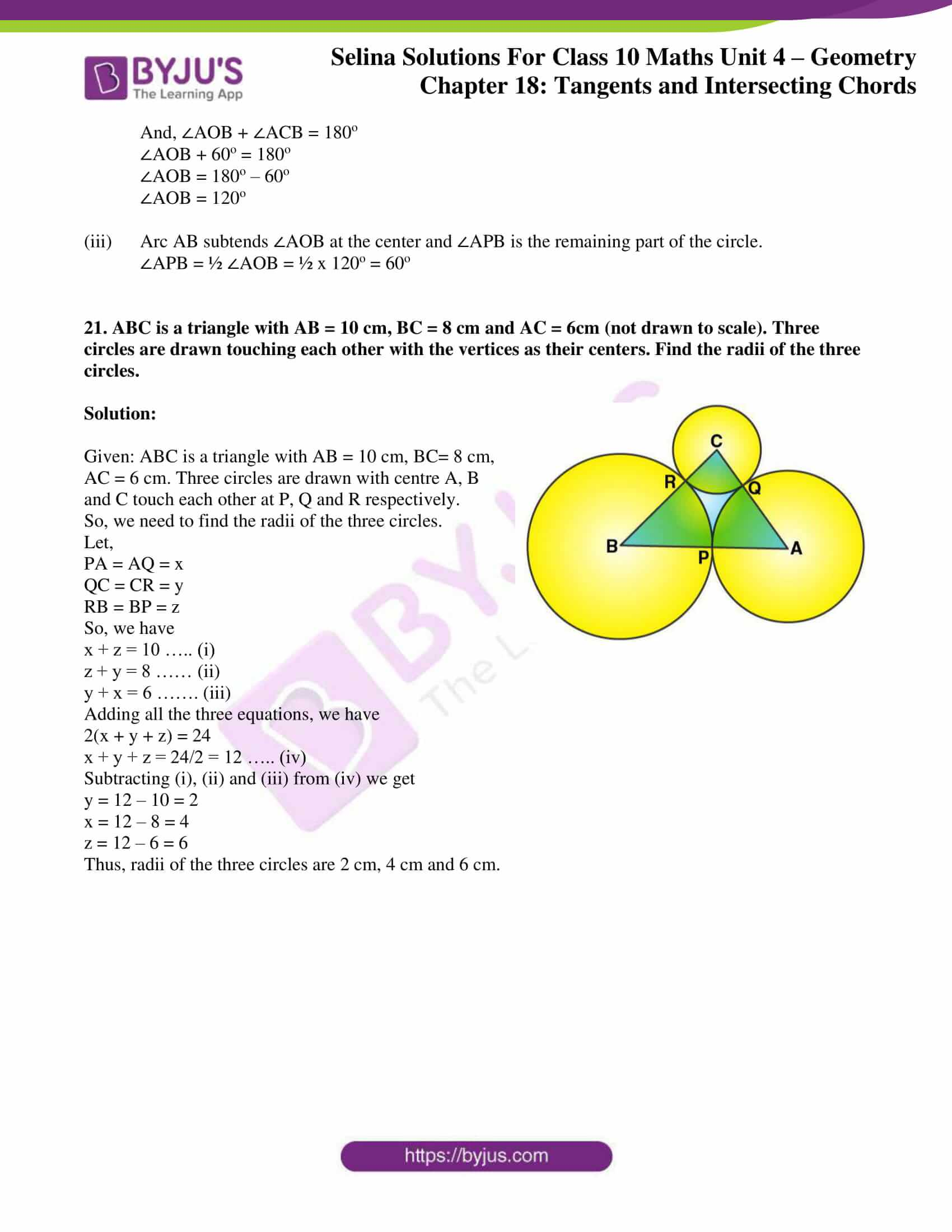### Access Selina Solutions Concise Maths Class 10 Chapter 18 Tangents and Intersecting Chords Exercise 18(C)

1. Prove that, of any two chords of a circle, the greater chord is nearer to the center.

Solution: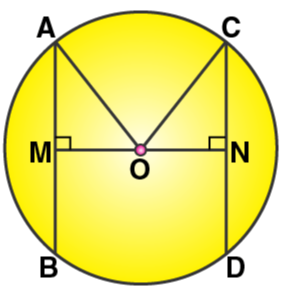Given: A circle with center O and radius r. AB and CD are two chords such that AB > CD. Also, OM ⊥ AB and ON ⊥ CD.

Required to prove: OM < ON

Proof:

Join OA and OC.

Then in right ∆AOM, we have

AO2 = AM2 + OM2

r2 = (½AB)2 + OM2

r2 = ¼ AB2 + OM2 ….. (i)

Again, in right ∆ONC, we have

OC2 = NC2 + ON2

r2 = (½CD)2 + ON2

r2 = ¼ CD2 + ON2 ….. (ii)

On equating (i) and (ii), we get

¼ AB2 + OM2 = ¼ CD2 + ON2

But, AB > CD [Given]

So, ON will be greater than OM to be equal on both sides.

Thus,

OM < ON

Hence, AB is nearer to the centre than CD.

2. OABC is a rhombus whose three vertices A, B and C lie on a circle with centre O.

i) If the radius of the circle is 10 cm, find the area of the rhombus.

ii) If the area of the rhombus is 32√3 cm2, find the radius of the circle.

Solution: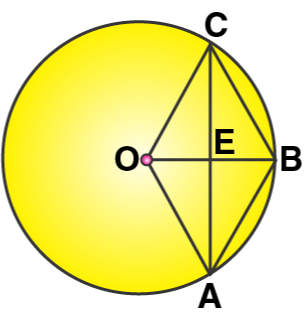(i) Given, radius = 10 cm

In rhombus OABC,

OC = 10 cm

So,

OE = ½ x OB = ½ x 10 = 5 cm

Now, in right ∆OCE

OC2 = OE2 + EC2

102 = 52 + EC2

EC2 = 100 – 25 = 75

EC = √75 = 5√3

Hence, AC = 2 x EC = 2 x 5√3 = 10√3

We know that,

Area of rhombus = ½ x OB x AC

= ½ x 10 x 10√3

= 50√3 cm2 ≈ 86.6 cm2

(ii) We have the area of rhombus = 32√3 cm2

But area of rhombus OABC = 2 x area of ∆OAB

Area of rhombus OABC = 2 x (√3/4) r2

Where r is the side of the equilateral triangle OAB.

2 x (√3/4) r2 = 32√3

√3/2 r2 = 32√3

r2 = 64

r = 8

Therefore, the radius of the circle is 8 cm.

3. Two circles with centers A and B, and radii 5 cm and 3 cm, touch each other internally. If the perpendicular bisector of the segment AB meets the bigger circle in P and Q; find the length of PQ.

Solution: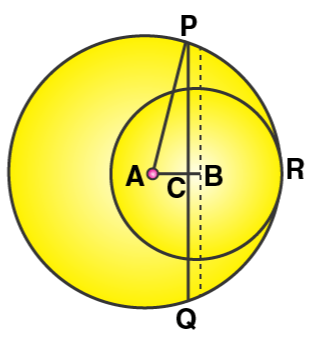We know that,

If two circles touch internally, then distance between their centres is equal to the difference of their radii. So, AB = (5 – 3) cm = 2 cm.

Also, the common chord PQ is the perpendicular bisector of AB.

Thus, AC = CB = ½ AB = 1 cm

In right ∆ACP, we have

AP2 = AC2 + CP2 [Pythagoras Theorem]

52 = 12 + CP2

CP2 = 25 – 1 = 24

CP = √24 cm = 2√6 cm

Now,

PQ = 2 CP

= 2 x 2√6 cm

= 4√6 cm

Therefore, the length of PQ is 4√6 cm.

4. Two chords AB and AC of a circle are equal. Prove that the center of the circle, lies on the bisector of the angle BAC.

Solution: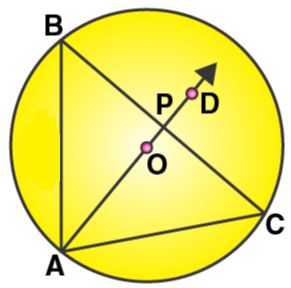Given: AB and AC are two equal chords of C (O, r).

Required to prove: Centre, O lies on the bisector of ∠BAC.

Construction: Join BC. Let the bisector of ∠BAC intersects BC in P.

Proof:

In ∆APB and ∆APC,

AB = AC [Given]

∠BAP = ∠CAP [Given]

AP = AP [Common]

Hence, ∆APB ≅ ∆APC by SAA congruence criterion

So, by CPCT we have

BP = CP and ∠APB = ∠APC

And,

∠APB + ∠APC = 180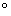[Linear pair]

2∠APB = 180[∠APB = ∠APC]

∠APB = 90Now, BP = CP and ∠APB = 90Therefore, AP is the perpendicular bisector of chord BC.

Hence, AP passes through the centre, O of the circle.

5. The diameter and a chord of circle have a common end-point. If the length of the diameter is 20 cm and the length of the chord is 12 cm, how far is the chord from the center of the circle?

Solution: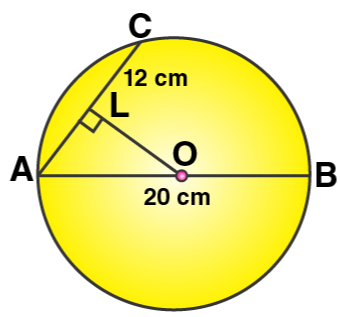We have, AB as the diameter and AC as the chord.

Now, draw OL ⊥ AC

Since OL ⊥ AC and hence it bisects AC, O is the centre of the circle.

Therefore, OA = 10 cm and AL = 6 cm

Now, in right ∆OLA

AO2 = AL+ OL2 [By Pythagoras Theorem]

102 = 62 + OL2

OL2 = 100 – 36 = 64

OL = 8 cm

Therefore, the chord is at a distance of 8 cm from the centre of the circle.

6. ABCD is a cyclic quadrilateral in which BC is parallel to AD, angle ADC = 110o and angle BAC = 50o. Find angle DAC and angle DCA.

Solution: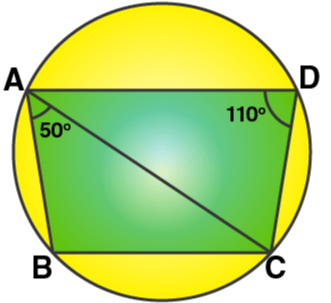And, ∠ADC = 110o, ∠BAC = 50o

We know that,

∠B + ∠D = 180o [Sum of opposite angles of a quadrilateral]

∠B + 110o = 180o

So, ∠B = 70o

∠BAC + ∠ABC + ∠ACB = 180o

50o + 70o + ∠ACB = 180o

∠ACB = 180o – 120o = 60o

And, as AD || BC we have

∠DAC = ∠ACB = 60o [Alternate angles]

∠DAC + ∠ADC + ∠DCA = 180o

60o + 110o + ∠DCA = 180o

Thus,

∠DCA = 180o – 170o = 10o

7. In the given figure, C and D are points on the semi-circle described on AB as diameter.

Given angle BAD = 70o and angle DBC = 30o, calculate angle BDC.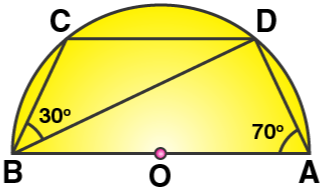Solution:

As ABCD is a cyclic quadrilateral, we have[Opposite angles of a cyclic quadrilateral are supplementary]

∠BCD + 70o = 180∠BCD = 180o – 70o = 110o

And, by angle sum property of ∆BCD we have

∠CBD + ∠BCD + ∠BDC = 180o

30o + 110o + ∠BDC = 180o

∠BDC = 180o – 140o

Thus,

∠BDC = 40o

8. In cyclic quadrilateral ABCD, A = 3 C and D = 5 B. Find the measure of each angle of the quadrilateral.

Solution: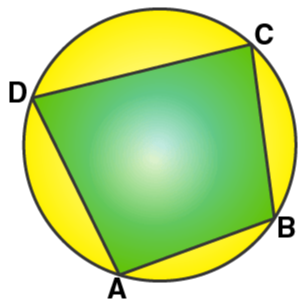So, ∠A + ∠C = 180o [Opposite angles in a cyclic quadrilateral is supplementary]

3∠C + ∠C = 180o [As ∠A = 3 ∠C]

∠C = 45o

Now,

∠A = 3 ∠C = 3 x 45o

∠A = 135o

Similarly,

∠B + ∠D = 180[As ∠D = 5 ∠B]

∠B + 5∠B = 180o

6∠B = 180o

∠B = 30o

Now,

∠D = 5∠B = 5 x 30o

∠D = 150o

Therefore,

∠A = 135o, ∠B = 30o, ∠C = 45o, ∠D = 150o

9. Show that the circle drawn on any one of the equal sides of an isosceles triangle as diameter bisects the base.

Solution: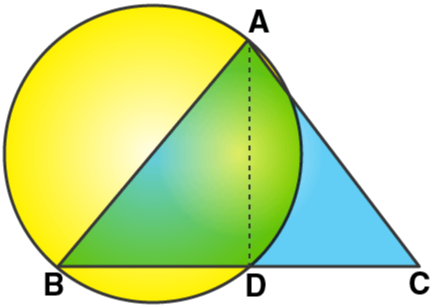And. AB is the diameter.

We have ∠ADB = 90º  [Angle in a semi-circle]

But,

Now, in ∆ABD and ∆ACD we have

AB = AC [Given]

Hence, ∆ABD ≅ ∆ACD by RHS congruence criterion

So, by C.P.C.T

BD = DC

Therefore, the circle bisects base BC at D.

10. Bisectors of vertex angles A, B and C of a triangle ABC intersect its circumcircle at points D, E and F respectively. Prove that angle EDF = 90o – ½ ∠A

Solution: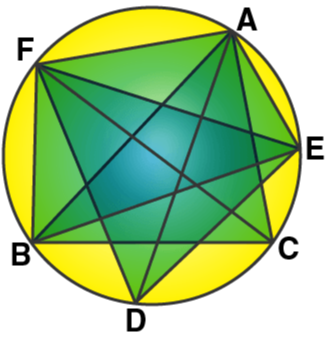Join ED, EF and DF. Also join BF, FA, AE and EC.

∠EBF = ∠ECF = ∠EDF ….. (i) [Angle in the same segment]

∠EBF + ∠EAF = 180o …… (ii)

[Sum of opposite angles in a cyclic quadrilateral is supplementary]

∠EAF + ∠ECF = 180o ……. (iii)

Adding (ii) and (iii) we get,

∠EBF + ∠ECF + 2∠EAF = 360o

∠EDF + ∠EDF + 2∠EAF = 360o [From (i)]

∠EDF + ∠EAF = 180o

∠EDF + ∠1 + ∠BAC + ∠2 = 180o

But, ∠1 = ∠3 and ∠2 and ∠4 [Angles in the same segment]

∠EDF + ∠3 + ∠BAC + ∠4 = 180o

But, ∠4 = ½ ∠C, ∠3 = ½ ∠B

Thus, ∠EDF + ½ ∠B + ∠BAC + ½ ∠C = 180o

∠EDF + ½ ∠B + 2 x ½ ∠A + ½ ∠C = 180o

∠EDF + ½ (∠A + ∠B + ∠C) + ½ ∠A = 180o

∠EDF + ½ (180o) + ½ ∠A = 180o

∠EDF + 90o + ½ ∠A = 180o

∠EDF = 180o – (90o + ½ ∠A)

∠EDF = 90o – ½ ∠A

11. In the figure, AB is the chord of a circle with centre O and DOC is a line segment such that BC = DO. If ∠C = 20o, find angle AOD.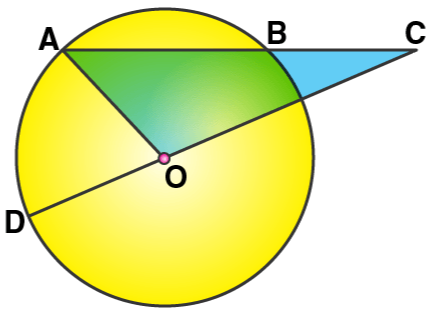Solution: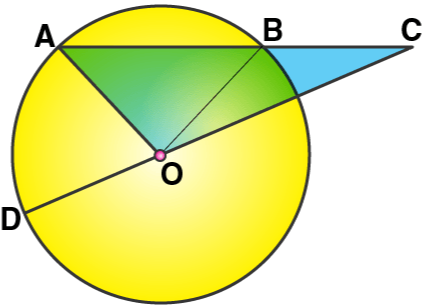Join OB.

In ∆OBC, we have

BC = OD = OB [Radii of the same circle]

∠BOC = ∠BCO = 20o

And ext. ∠ABO = ∠BCO + ∠BOC

Ext. ∠ABO = 20o + 20o = 40o ….. (1)

Now in ∆OAB,

OA = OB [Radii of the same circle]

∠OAB = ∠OBA = 40o [from (1)]

∠AOB = 180o – 40o – 40o = 100o

As DOC is a straight line,

∠AOD + ∠AOB + ∠BOC = 180o

∠AOD + 100o + 20o = 180o

∠AOD = 180o – 120o

Thus, ∠AOD = 60o

12. Prove that the perimeter of a right triangle is equal to the sum of the diameter of its incircle and twice the diameter of its circumcircle.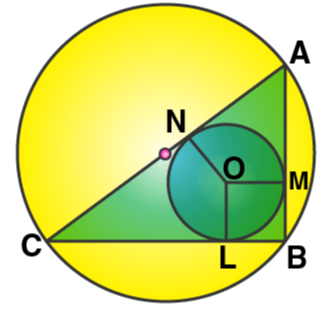Solution:

Let’s join OL, OM and ON.

And, let D and d be the diameter of the circumcircle and incircle.

Also, let R and r be the radius of the circumcircle and incircle.

Now, in circumcircle of ∆ABC,

∠B = 90o

Thus, AC is the diameter of the circumcircle i.e. AC = D

Let the radius of the incircle be ‘r’

OL = OM = ON = r

Now, from B, BL and BM are the tangents to the incircle.

So, BL = BM = r

Similarly,

AM = AN and CL = CN = R

[Tangents from the point outside the circle]

Now,

AB + BC + CA = AM + BM + BL + CL + CA

= AN + r + r + CN + CA

= AN + CN + 2r + CA

= AC + AC + 2r

= 2AC + 2r

= 2D + d

– Hence Proved

13. P is the midpoint of an arc APB of a circle. Prove that the tangent drawn at P will be parallel to the chord AB.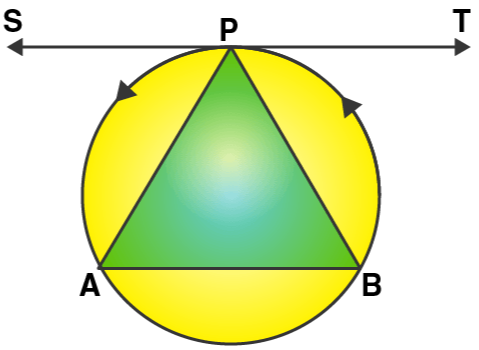Solution:

First join AP and BP.

As TPS is a tangent and PA is the chord of the circle.

∠BPT = ∠PAB  [Angles in alternate segments]

But,

∠PBA = ∠PAB [Since PA = PB]

Thus, ∠BPT = ∠PBA

But these are alternate angles,

Hence, TPS || AB

14. In the given figure, MN is the common chord of two intersecting circles and AB is their common tangent.

Prove that the line NM produced bisects AB at P.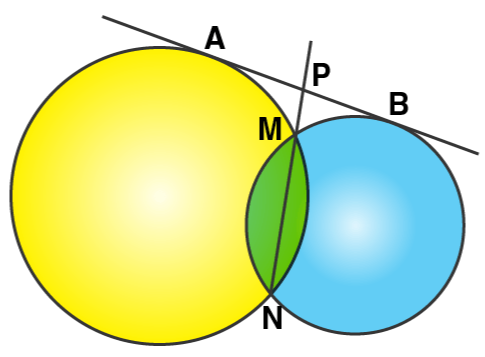Solution:

From P, AP is the tangent and PMN is the secant for first circle.

AP2 = PM x PN …. (1)

Again from P, PB is the tangent and PMN is the secant for second circle.

PB2 = PM x PN …. (2)

From (i) and (ii), we have

AP2 = PB2

AP = PB

Thus, P is the midpoint of AB.

15. In the given figure, ABCD is a cyclic quadrilateral, PQ is tangent to the circle at point C and BD is its diameter. If ∠DCQ = 40o and ∠ABD = 60o, find:

i) ∠DBC

ii) ∠BCP

Solution: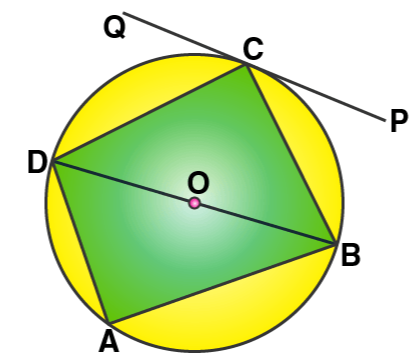PQ is a tangent and CD is a chord.

∠DCQ = ∠DBC [Angles in the alternate segment]

∠DBC = 40o [As ∠DCQ = 40o]

(ii) ∠DCQ + ∠DCB + ∠BCP = 180o

40o + 90o + ∠BCP = 180o [As ∠DCB = 90o]

∠BCP = 180o – 130o = 50o

(iii) In ∆ABD,

∠BAD = 90o [Angle in a semi-circle], ∠ABD = 60o [Given]

∠ADB = 180o – (90o + 60o)

∠ADB = 180o – 150o = 30o

16. The given figure shows a circle with centre O and BCD is a tangent to it at C. Show that: ∠ACD + ∠BAC = 90o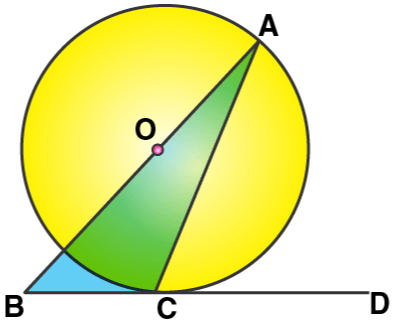Solution: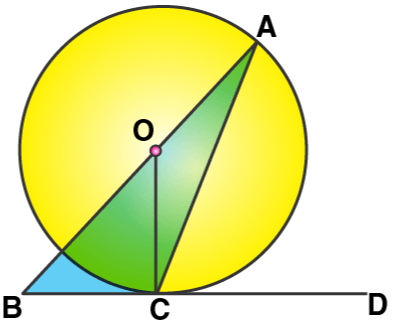Let’s join OC.

BCD is the tangent and OC is the radius.

As, OC ⊥ BD

∠OCD = 90o

∠OCD + ∠ACD = 90o …. (i)

But, in ∆OCA

OA = OC [Radii of the same circle]

Thus, ∠OCA = ∠OAC

Substituting in (i), we get

∠OAC + ∠ACD = 90o

Hence, ∠BAC + ∠ACD = 90o

17. ABC is a right triangle with angle B = 90º. A circle with BC as diameter meets by hypotenuse AC at point D. Prove that:

i) AC x AD = AB2

ii) BD2 = AD x DC.

Solution: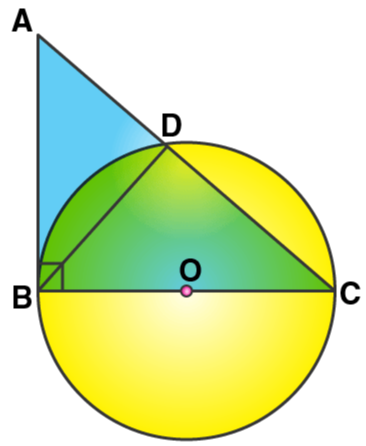i) In ∆ABC, we have

∠B = 90o and BC is the diameter of the circle.

Hence, AB is the tangent to the circle at B.

Now, as AB is tangent and ADC is the secant we have

∠D = 90o

So, ∠A + ∠ABD = 90o …… (i)

But in ∆ABC, ∠B = 90o

∠A + ∠C = 90o ……. (ii)

From (i) and (ii),

∠C = ∠ABD

Now in ∆ABD and ∆CBD, we have

∠BDA = ∠BDC = 90o

∠ABD = ∠BCD

Hence, ∆ABD ~ ∆CBD by AA postulate

So, we have

Therefore,

18. In the given figure, AC = AE.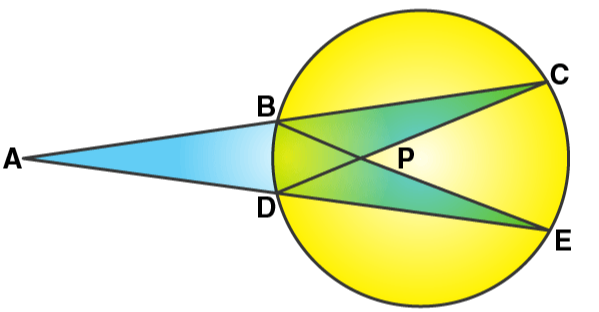Show that:

i) CP = EP

ii) BP = DP

Solution:

∠ACD = ∠AEB  [Angles in the same segment]

AC = AE [Given]

∠A = ∠A [Common]

Hence, ∆ADC ≅ ∆ABE by ASA postulate

So, by C.P.C.T we have

But, AC = AE [Given]

So, AC – AB = AE – AD

BC = DE

In ∆BPC and ∆DPE,

∠C = ∠E  [Angles in the same segment]

BC = DE

∠CBP = ∠CDE [Angles in the same segment]

Hence, ∆BPC ≅ ∆DPE by ASA postulate

So, by C.P.C.T we have

BP = DP and CP = PE

19. ABCDE is a cyclic pentagon with centre of its circumcircle at point O such that AB = BC = CD and angle ABC = 120o

Calculate:

i) ∠BEC

ii) ∠BED

Solution: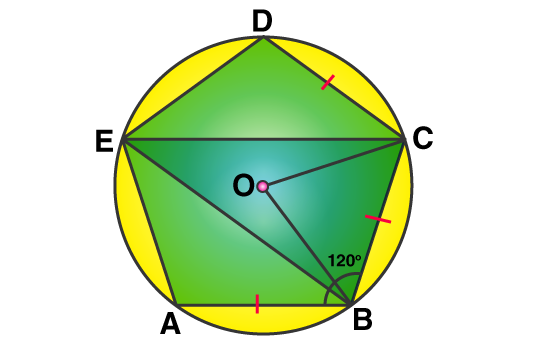i) Join OC and OB.

AB = BC = CD and ∠ABC = 120o [Given]

So, ∠BCD = ∠ABC = 120o

OB and OC are the bisectors of ∠ABC and ∠BCD and respectively.

So, ∠OBC = ∠BCO = 60o

In ∆BOC,

∠BOC = 180o – (∠OBC + ∠BOC)

∠BOC = 180o – (60o + 60o) = 180o – 120o

∠BOC = 60o

Arc BC subtends ∠BOC at the centre and ∠BEC at the remaining part of the circle.

∠BEC = ½ ∠BOC = ½ x 60o = 30o

ii) In cyclic quadrilateral BCDE, we have

∠BED + ∠BCD = 180o

∠BED + 120o = 180o

Thus, ∠BED = 60o

20. In the given figure, O is the centre of the circle. Tangents at A and B meet at C. If angle ACO = 30o, find:

(i) angle BCO

(ii) angle AOB

(iii) angle APB

Solution: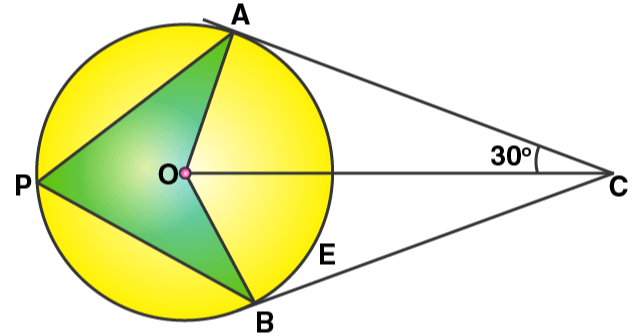In the given fig, O is the centre of the circle and, CA and CB are the tangents to the circle from C. Also, ∠ACO = 30o

P is any point on the circle. P and B are joined.

To find:

(i) ∠BCO

(ii) ∠AOB

(iii) ∠APB

Proof:

(i) In ∆OAC and ∆OBC, we have

OC = OC [Common]

OA = OB [Radii of the same circle]

CA = CB [Tangents to the circle]

Hence, ∆OAC ≅ ∆OBC by SSS congruence criterion

Thus, ∠ACO = ∠BCO = 30o

(ii) As ∠ACB = 30o + 30o = 60o

And, ∠AOB + ∠ACB = 180o

∠AOB + 60o = 180o

∠AOB = 180o – 60o

∠AOB = 120o

(iii) Arc AB subtends ∠AOB at the center and ∠APB is the remaining part of the circle.

∠APB = ½ ∠AOB = ½ x 120o = 60o

21. ABC is a triangle with AB = 10 cm, BC = 8 cm and AC = 6cm (not drawn to scale). Three circles are drawn touching each other with the vertices as their centers. Find the radii of the three circles.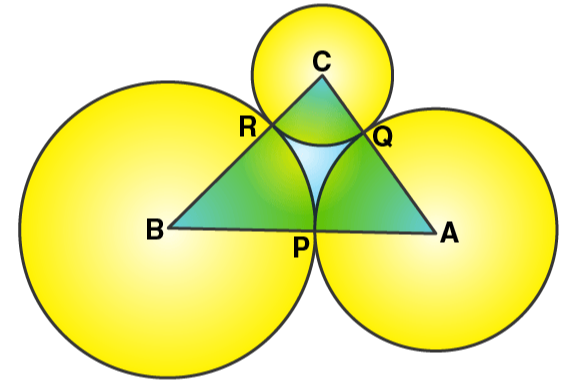Solution:

Given: ABC is a triangle with AB = 10 cm, BC= 8 cm, AC = 6 cm. Three circles are drawn with centre A, B and C touch each other at P, Q and R respectively.

So, we need to find the radii of the three circles.

Let,

PA = AQ = x

QC = CR = y

RB = BP = z

So, we have

x + z = 10 ….. (i)

z + y = 8 …… (ii)

y + x = 6 ……. (iii)

Adding all the three equations, we have

2(x + y + z) = 24

x + y + z = 24/2 = 12 ….. (iv)

Subtracting (i), (ii) and (iii) from (iv) we get

y = 12 – 10 = 2

x = 12 – 8 = 4

z = 12 – 6 = 6

Thus, radii of the three circles are 2 cm, 4 cm and 6 cm.